# Number Converter Extension-Decimal,Binary,Hexadecimal and Octal Conversion

Number Converter

### Write a short description

My second extension, Number Converter helps you for the conversion of binary, decimal, hexadecimal and octal number into any of this type.

### Add a picture of all the blocks

1) To Convert Decimal Number to Binary Number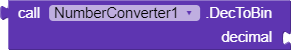It helps you to convert Decimal Number to Binary Number. Remember It always accepts Integer value.

2) To Convert Decimal Number to Octal Number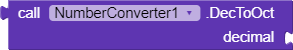It helps you to convert Decimal Number to Octal Number. It always accepts Integer value.

3) To Convert Decimal Number to Hexadecimal Number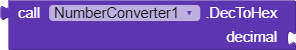It helps you to convert Decimal Number to Hexadecimal Number. It always accepts Integer value.

4) To Convert Binary Number To Decimal Number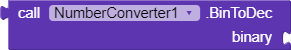It helps you to convert Binary Number to Decimal Number. It always accepts String Value.

5)To Convert Binary Number To Octal Number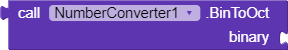It helps you to convert Binary Number toOctal Number. It always accepts String Value.

6)To Convert Binary Number To Hexadecimal Number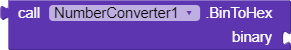It helps you to convert Binary Number to Hexadecimal Number. It always accepts String Value.

7)To Convert Octal To Decimal Number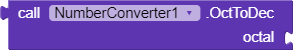It helps you to convert Octal Number to Decimal Number. It always accepts String Value.

8) To Convert Octal To Binary Number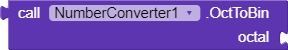It helps you to convert Octal Number to Binary Number. It always accepts String Value.

9)To Convert Octal Number To Hexadecimal Number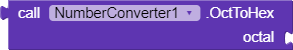It helps you to convert Octal Number to Hexadecimal Number. It always accepts String Value.

10)Convert Hexadecimal Number To Decimal Number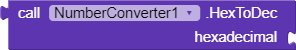It helps you to convert Hexadecimal Number to Decimal Number. It always accepts String Value.

11)Convert Hexadecimal Number To Binary Number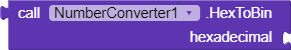It helps you to convert Hexadecimal Number to Binary Number. It always accepts String Value.

12)Convert Hexadecimal Number To Octal Number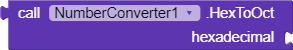It helps you to convert Hexadecimal Number to Octal Number. It always accepts String Value.

### Instructions to use this extension:

You should always know that Decimal Number always accept Integer value and Other Number Systems except for Decimal Number always accept string value. For eg;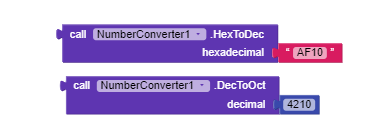Here, hexadecimal has accepted text value ie string and decimal has accepted math value ie integer value.

Thanks to Kodular For This Amazing Platform//Sneha

16 Likes

Very nice @oseamiya Keep Developing. Keep Kodingthis extension does not have the ability to translate HextoDEX numbers and vice versa, TAKING INTO ACCOUNT the BITNESS of the number. For example, the number FFFF in bit depth 16 is -1, and in bit depth 32 it is 65535. Without the ability to control the HEX bit depth, this add-on is not so popular.

Hi, thank you so much for creating this extension

and umm… actually, I just recently started learning Kodular and if it’s not too much bother to ask… could you possibly show me the practical use of this extension?

Thank You~